Jacobi condition

A necessary condition for optimality in problems in the calculus of variations. The Jacobi condition is a necessary condition for the second variation of a functional being minimized to be non-negative at a point where it is minimal (the vanishing of the first variation of the functional is ensured by the first-order necessary condition: the Euler equation, the transversality condition and the Weierstrass conditions (for a variational extremum)).

One poses the problem of minimizing, for example, the functional(1)

under given conditions at the end points:(2)

If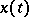,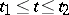, is a solution to the problem (1), (2), then the first variation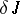of the functional must vanish, and so one obtains the first-order necessary conditions, and the second variation(3)

must be greater than or equal to 0 for any piecewise-smooth function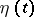satisfying the zero boundary conditions: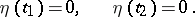(4)

The Euler equation for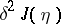is: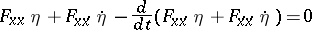(5)

and is called the Jacobi equation. It is a second-order linear differential equation in the unknown function. All the coefficients ofand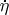in (5) are evaluated at the values of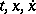corresponding to a known optimal solution, and so they are all known functions of.

The function,, satisfies the Jacobi equation under the boundary conditions (4), that is, it is an extremal of. On the other hand, for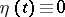the second variation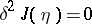, and since for an optimal solutionthe second variation is non-negative for any, the function,, minimizes. If Legendre's condition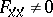,, holds (cf. also Legendre condition), that is,is a non-singular extremal, then under the initial conditions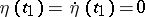, the solution to the Jacobi equation is identically zero.

A point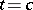is called conjugate to a point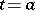if there is a solution to the Jacobi equation that vanishes atand, and is not identically zero betweenand. By the necessary Jacobi condition, if a non-singular extremal,, gives a minimum of the functional (1), thendoes not contain points conjugate to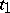.

The practical meaning of the Jacobi condition can be explained as follows. Suppose that it does not hold, that is, there is a point,, conjugate to. Then one can construct the continuous function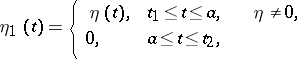that is a solution to (5) for which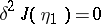. Thus,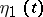,, is a polygonal extremal ofwith a corner at. But by the necessary condition of Weierstrass–Erdmann (see Euler equation), which requires the continuity of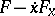andat the corner, atone must have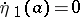. This, together with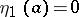, gives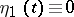, in contradiction to the assumption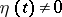,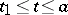.

To verify the Jacobi condition directly one has to consider the solution to (5) that satisfies the initial conditionsLet it be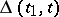. For a point,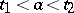, to be conjugate toit is necessary and sufficient that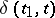vanishes at. Hence, the fulfilment of the Jacobi condition is equivalent to the non-vanishing ofon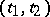.

In a more general case, when a variational problem (a problem in Lagrange's, Mayer's or Bolza's form) is being considered, the statement of the Jacobi condition has certain special features. The problem of minimizing the second variation of the functional is stated as a Bolza problem. This problem is called the associated problem, and its extremals are called associated extremals. The differential conditions of the constraint, and the boundary conditions in the associated problem of minimizing the second variation, are obtained as a result of variation of the corresponding conditions of the original variational problem. The form of the definition of a conjugate point remains the same. For the second variation of the functional to be non-negative on the class of associated extremals satisfying the associated condition at the end points, the Jacobi condition must hold; this requires thatdoes not contain points conjugate to.

The Jacobi condition was established by C.G.J. Jacobi (1837).